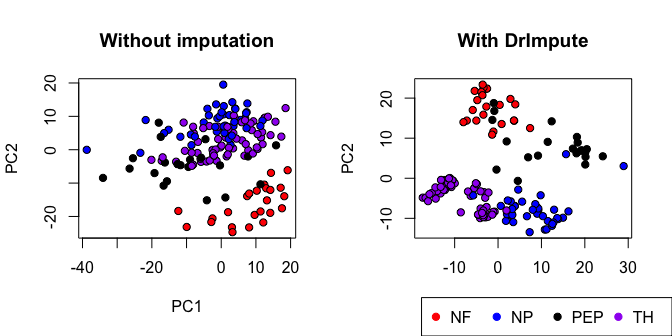# DrImpute : imputing dropout events in single-cell RNA-sequencing data

#### 2017-07-15

This vignette illustrates the use of DrImpute software in single cell RNA sequencing data analysis.

## Data preparation

Example data is taken from Usoskin et al. (2015), GSE59739. We randomly selected 150 cells from original 799 cells.

Firstly, genes that are expressed less than 2 cells are removed.

data(exdata)
exdata <- preprocessSC(exdata)
## ----------------------------------------------------------------
## Preprocess single cell RNA-seq expression matrix
## ----------------------------------------------------------------
## number of input genes(nrow(X))=25334
## number of input cells(ncol(X))=150
## number of input cells that express at least 0 genes=150
## number of input genes that are expressed in at least 2 cells and at most 100% cells=13704
## sparsity of expression matrix=74.5%

Normalization is performed using total read count for simplicity, and then log transformation is applied.

sf <- apply(exdata, 2, mean)
npX <- t(t(exdata) / sf )
lnpX <- log(npX+1)

## Data analysis

Dropout Imputation can be simply done using DrImpute function.

lnpX_imp <- DrImpute(lnpX)
## Calculating Spearman distance.
## Calculating Pearson distance.
##  Clustering for k : 10
##  Clustering for k : 11
##  Clustering for k : 12
##  Clustering for k : 13
##  Clustering for k : 14
##  Clustering for k : 15
## cls object have 12 number of clustering sets.
##
##
##  Zero percentage :
## Before impute : 75 percent.
## After impute : 17 percent.
## 57 percent of zeros are imputed.

The ratio of zero is 0.75, and 57 percent of zero’s are imputed by DrImpute.

We visualized single cell RNA sequencing data using PCA with and without imputation by DrImpute.

## Loading required package: MatrixPrior to the use of DrImpute, the NP, TH, and PEP groups are visually indistinguishable in the 2D space. However, after using DrImpute, NP, TH, and PEP have better separation.